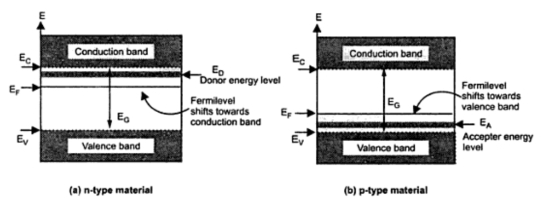### Fermi Level in Extrinsic Semiconductor

When the impurities are added to the intrinsic semiconductor the allowable energy levels are introduced and material becomes extrinsic semiconductor.
In n-type semiconductor, a donor impurity is added. Each donor atom donates one free electron and there are large number of free electrons, available in the conduction band. The donor energy level corresponding to the donor impurity added is just below the conduction band. This donor level is indicated as ED and its distance is 0.01 eV below the conduction band in germanium while it is 0.05 eV below the conduction band in silicon. As this distance is very small, even at room temperature, almost all the extra electrons from the donor impurity atoms jump into the conduction band. Hence number of free electrons is very large in case of n-type material. Due to abundant free electrons, the probability of occupying the energy level by the electrons, towards the conduction band is more. This probability is indicated by Fermi level EF. So in n-type material, the fermi level EF gets shifted towards the conduction band. But it is below the donor energy level. The overall energy band diagram for n-type material is shown in the Fig. 1(a).Fig. 1  Energy band diagrams for extrinsic semicondcutor
As against this, in p-type material, acceptor impurity is added. Due to this, large number of holes get created in the valence band. The acceptor energy level corresponding to acceptor impurity gets introduced which is indicated as EA and is very close to the valence band just above it. At room temperature, the electrons from valence band jump tp acceptor energy level, leaving behind the holes in valence band. This shifts the fermi level EF towards the valence band. It lies above the acceptor energy level. The overall energy band diagram for p-type material is shown in the Fig. 1(b).
Note : It must be noted that the doped material is always electrically neutral as the total number of electrons is equal to the total number of protons, after the addition of impurity.